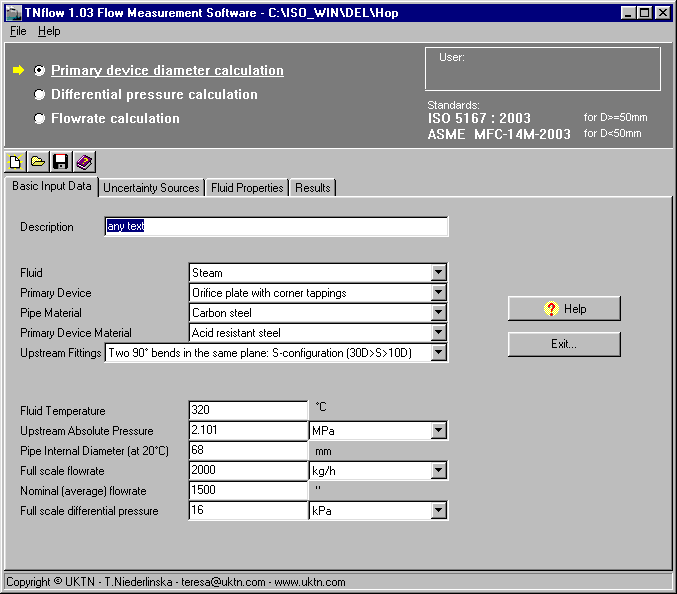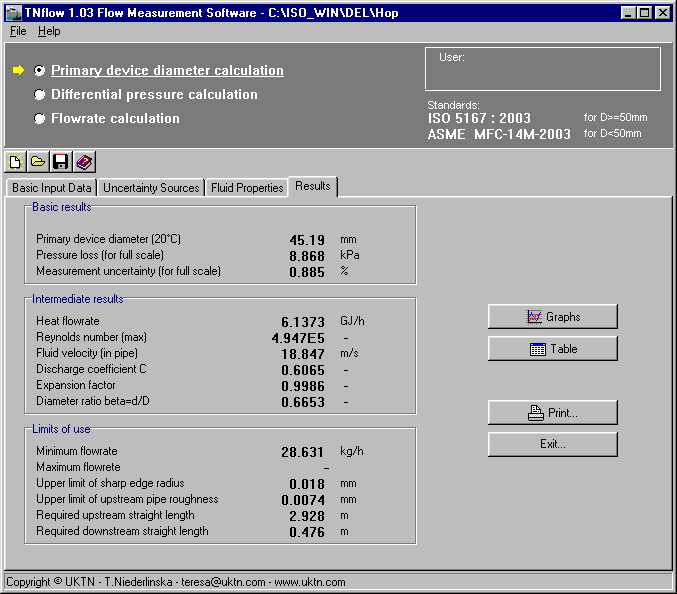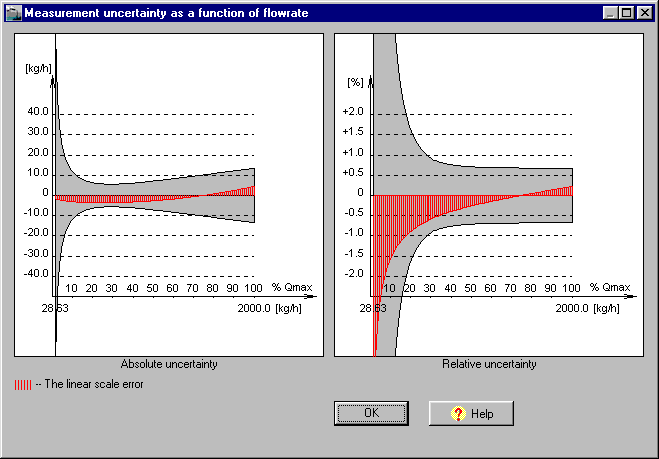uktn Uslugi KomputeroweTeresa Niederlinska 44-100 Gliwice, Polandul. Pszczynska 118A/28 teresa@uktn.com

(May, 2023)

#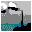TNflow - Flow  Measurement  Software based on:

## ISO 5167:2022ASME MFC-14M-2003ISO/TR 15377:2018

Windows

TNflow is a stand-alone software.
TNflow is addressing the needs of engineers dealing with fluid flow measurement by means of pressure differential
devices.
TNflow may be used to calculate:

1. primary device diameter (bore diameter) for declared full scale flowrate and related value of differential pressure,
2. differential pressure for declared bore diameter and flowrate,
3. flowrate for declared bore diameter and differential pressure.

Any of the above calculations is supplemanted by:

• diameters corrections for thermal expansion,
• bounds of measurement range calculations,
• upper limit of upstream pipe roughness calculations,
• upper limit of sharp edge radius calculations,
• required upstream and downstream straight lengths calculations,
• uncertainty analysis of flowrate measurement at a single point and in the entire measurement range,
• bias "linear scale" error analysis.

The calculations may be performed for following orifice plates, nozzles, Venturi nozzles and Venturi tubes covered by
ISO 5167:2022 and ISO/TR 15377:2018 :

TNflow calculates fluid properties for the most common fluids:

• water and steam (The 1967 IFC Formulation for Industrial Use,  The IAPS Formulation 1985 for Viscosity),
• natural gas (ISO 12213-3, GOST 30319.1-96),
• coke-oven or town gas (Beate-Bridgeman state equation),
• air (Redlich-Kwong state equation),
• other gas if its composition is known (Redlich-Kwong state equation),
• liquid ammonia (Raznievic).

For other fluids the properties may be declared by user.

## Graphs of uncertainty and bias "linear scale" error

TNflow displays the graphs of the uncertainty and of the "linear scale" error for the entire measurement range.
The bias "linear scale" error is a difference between values of flowrate calculated using accurate method: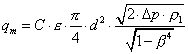(where C and E are functions of q),

and values of flowrate calculated using simplified method: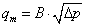(where B is constant for the entire measurement range).

Click on an image to see the full size picture: